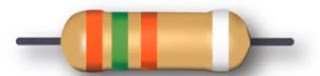## Sunday, September 19, 2021

WHAT IS VFD AND HOW IT WORKS?

### WHAT IS VFD?

A variable frequency drive (VFD) is a motor control device that protects and controls the speed of an AC Inductor motor.VFD can also control the speed of the motor during time of the start and stop cycle, as well as throughout the run cycle .

### HOW DOES VFD WORK?

It converts input power to adjustable frequency and voltage source for controlling speed of AC induction motors. The frequency of the power applied to an AC motor determines the motor speed, based on the formula –

N = (120 X F) / P

Here – N =   Speed of rotor of induction motor (in RPM)

P =    Number of Pole

F =     Supply frequency

For example, A four pole motor is operating at 50 Hz .Now we will calculate the speed as per above formula –

N = (120 X F) / P

N = (120 X 50) /4

N = 1500 RPM

Now we will change the frequency at 40Hz then speed will be

N = (120 X 40) /4     = 1200RPM

Here we can see speed will be increase as per frequency change.

### MAIN PART OF VFD

1.  CONVERTER – The input section of the derive is the converter, It is built based on bridge rectifier which convert AC into DC.

2.  FILTER – Which smooth out the DC output of Converter, every DC output have some ripple so filter remove the ripple and get the pure DC output at Filter.

3.  INVERTOR – This section inverts the DC voltage back to AC

·         High starting torque

·         Protection (like short circuit, over load, earth leakage etc.)

·         Reduce power when not required

·         Smooth starting

·         Energy saving

·         Power factor improvement

·         Easily reversal motor

### APPLICATION OF VFD –

INDUSTRAIL APPLICATION

·         Use in conveyor system

·         Use in large scale machine tools industry for speed control of different motor.

·         Use in lift and escalator

·         Use in metro and railways

## WHAT IS RESISTANCE?

RESISTANCE is a material which opposes the flow of current in it. Resistance property is that have large number of atom due to this it oppose the flow of current in it.

What is the unit of RESISTANCE?

The Standard unit of RESISTANCE is OHM and symbol of R is use in circuit.

How to calculate the resistance in wire?

The resistance of a conducting wire is to be directly proportional to its length L.

The resistance of a conducting wire is to be inversely proportional to its area of cross section (A)

It is depend upon on temperature and nature of conducting material.

R L/A ,  R = pL/ A

Here is

·                  R =   Resistance of Wire or Conductor

·                  L =    Length of Conductor

·                  A =    Cross – section area of Conductor or wire

·                  P =    Resistivity of a material

### What is Resistivity?

Specific electrical resistance is called resistance, it is depend on the mow much strong material for oppose the flow of current, its depend on per unit length and per unit area and also specified particular temperature,

### How to measure color code of resistance?

Best known of color code of resistance have BBROY has Great Britain Is Very Good Wife,

1.      BLACK

2.      BROWN

3.      RED

4.      ORANGE

5.      YELOOW

6.      GREEN

7.      BLUE

8.      VIOLET

9.      GRAY

10.   WHITE

11.   GOLD

12.   SILVER

Here is an example to measure color code of resistance,As per shown image have colored bands of RED, GREEN, RED and SILVER.

Now we will use the formula is R = (1st digit x10+2nd digit) x Multiplier

R = (RED x 10 + GREEN) X RED

R = (2×10+5) x 100

R = 25 x 100 = 2500 Ohm = 2.5 K

Last Band Gold which is tolerance 10%